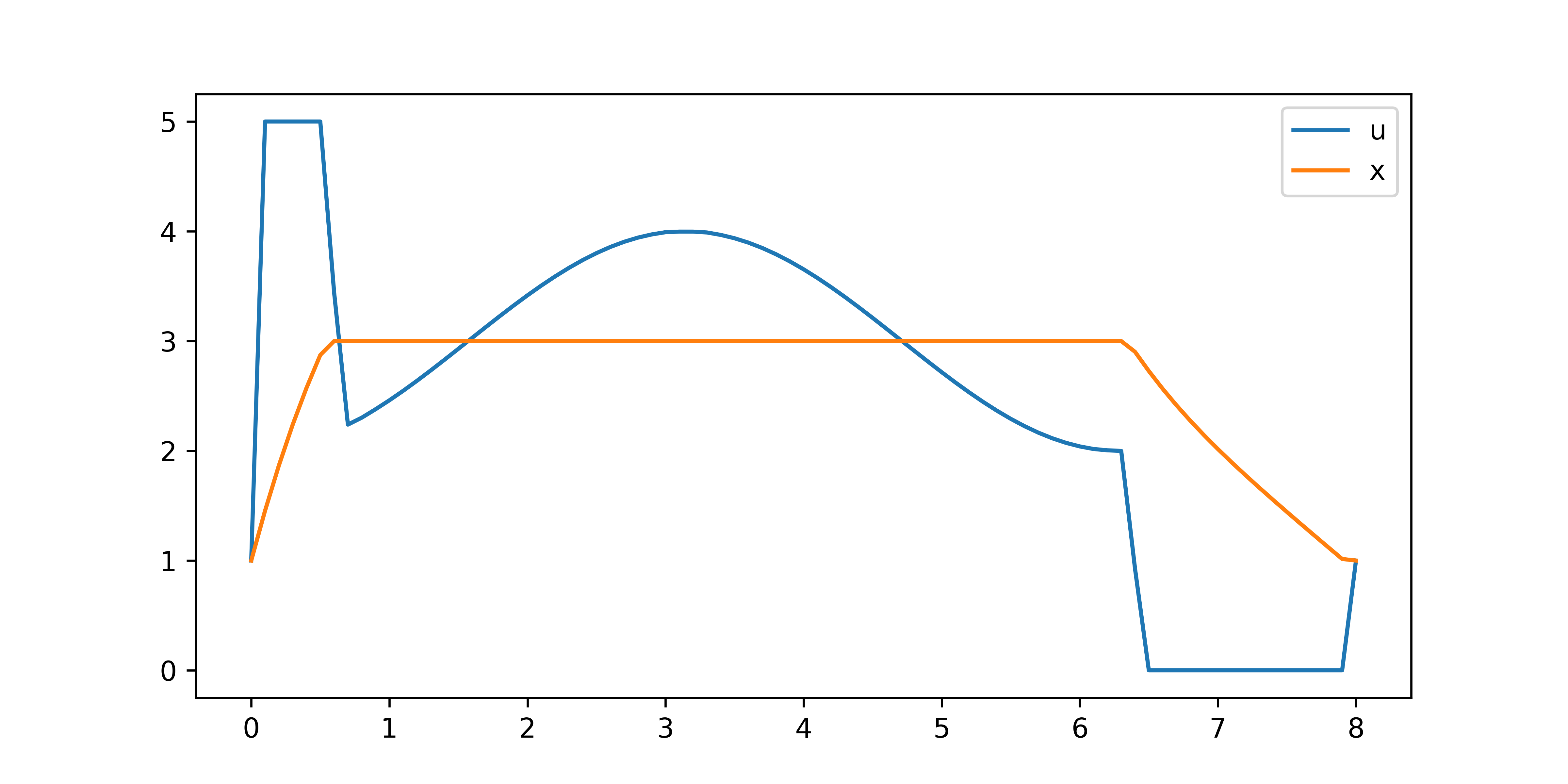### Periodic Boundary Conditions

Periodic boundary conditions arise in any situation where the end point must be equal to the beginning point. This type of boundary condition is typical where something is repeating many times but the optimization or simulation only needs to take place over one cycle of that sequence. An examples of a repeating process is the body's natural Circadian rhythm or a power plant that produces power to follow daily demand cycles. Examples of periodic boundary conditions in natural cycles or in manufacturing processes give importance to these conditions in numerical simulation.

Boundary conditions are added for select variables with the use of a periodic object declaration.

APMonitor Model

Objects
q = periodic
End Objects

In Python Gekko, there is a periodic function to add the APMonitor periodic condition.

Python Gekko

m.periodic(q)

Linking this periodic object to a variable in the model enforces the periodic condition by adding an additional equation that the end point must be equal to the beginning point in the horizon.

An example illustrates the use of periodic boundary conditions.

$$\min_u \left(x-3\right)^2$$

$$\frac{dx}{dt}+x=cos(t)+u$$

$$x(0)=x(8)=1$$

$$u(0)=u(8)=1$$

$$0 \le u \le 5$$from gekko import GEKKO
import numpy as np
m = GEKKO()
m.time = np.linspace(0,8,81)
t = m.Param(m.time)
u = m.MV(1,lb=0,ub=5); u.STATUS=1
x = m.Var(1)
m.periodic(u)
m.periodic(x)
m.Minimize((x-3)**2)
m.Equation(x.dt()+x==m.cos(t)+u)
m.options.IMODE = 6
m.solve()

import matplotlib.pyplot as plt
plt.plot(m.time,u,m.time,x)
plt.legend(['u','x'])
plt.show()

Scripts in MATLAB and Python are available below to recreate this solution along with the model equations in APMonitor. Both MATLAB and Python scripts produce equivalent results.Periodic Example Script in MATLAB/Python (zip file)

Objects
q = periodic
End Objects

Connections
s = q.x
End Connections

Constants
eps = 0.7
End Constants

Parameters
d
p
End Parameters

Variables
s >= 0 , = 100
stored
recovery
vx >= 0  # slack variable for energy storage representation
vy >= 0  # slack variable for energy recovery representation
End Variables

Equations
minimize p
p + recovery/eps - stored >= d
p - d = vx- vy
stored = p-d + vy
recovery = d- p + vx
$s = stored - recovery/ eps stored * recovery <= 0 End Equations File *.plt New Trend p s d End File #### Periodic Energy Storage A further example demonstrates a more complicated model for energy storage and retrieval. In this case, energy is stored during the first hours of the day when demand is lower. The power generation runs at a constant level while the energy storage is able to follow the cyclical demand. Energy storage is set to a periodic boundary condition to ensure that the beginning and end of the day have at least 100 units of stored energy. Scripts are available in both MATLAB and Python. #!/usr/bin/env python3 # -*- coding: utf-8 -*- """ Created on Mon Mar 8 21:34:49 2021 Gekko implementation of the simple energy storage model found here: https://www.sciencedirect.com/science/article/abs/pii/S030626191500402X Useful link: https://apmonitor.com/wiki/index.php/Apps/PeriodicBoundaryConditions @author: Nathaniel Gates, John Hedengren """ import numpy as np import matplotlib.pyplot as plt import matplotlib.ticker as mtick from gekko import GEKKO m = GEKKO(remote=False) t = np.linspace(0, 24, 24*3+1) m.time = t m.options.SOLVER = 1 m.options.IMODE = 6 m.options.NODES = 3 m.options.CV_TYPE = 1 m.options.MAX_ITER = 300 p = m.FV() # production p.STATUS = 1 s = m.Var(100, lb=0) # storage inventory store = m.SV() # store energy rate vy = m.SV(lb=0) # store slack variable recover = m.SV() # recover energy rate vx = m.SV(lb=0) # recover slack variable eps = 0.7 d = m.MV(-20*np.sin(np.pi*t/12)+100) m.periodic(s) m.Equations([p + recover/eps - store >= d, p - d == vx - vy, store == p - d + vy, recover == d - p + vx, s.dt() == store - recover/eps, store * recover <= 0]) m.Minimize(p) m.solve(disp=True) #%% Visualize results fig, axes = plt.subplots(4, 1, sharex=True) ax = axes ax.plot(t, store, 'C3-', label='Store Rate') ax.plot(t, recover, 'C0-.', label='Recover Rate') ax = axes ax.plot(t, d, 'k-', label='Electricity Demand') ax.plot(t, p, 'C3--', label='Power Production') ax = axes ax.plot(t, s, 'C2-', label='Energy Inventory') ax = axes ax.plot(t, vx, 'C2-', label='$S_1$') ax.plot(t, vy, 'C3--', label='$S_2\$')
ax.set_xlabel('Time (hr)')

for ax in axes:
ax.legend(bbox_to_anchor=(1.01, 0.5), \
loc='center left', frameon=False)
ax.grid()
ax.set_xlim(0, 24)
loc = mtick.MultipleLocator(base=6)
ax.xaxis.set_major_locator(loc)

plt.tight_layout()
plt.show()Periodic Energy Storage in MATLAB/Python (zip file)#### Reference

• Safdarnejad, S.M., Hedengren, J.D., Baxter, L.L., Plant-level dynamic optimization of Cryogenic Carbon Capture with conventional and renewable power sources, Applied Energy, Volume 149, 2015, Pages 354-366, ISSN 0306-2619, DOI: 10.1016/j.apenergy.2015.03.100. Article